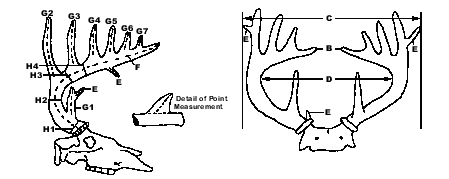If you have Excel 2000 on your computer, the Auto Score Sheet will automatically tally your whitetail's total scores if you enter each individual measurement.  This feature only works if you have Excel 2000.  If you don't have Excel 2000, use the manual score sheet below.

This is the scoring system for Whitetail and Coues' Deer.  This system can be used for Boone and Crockett, Pope and Young, typical and non-typical measurements.  All measurements must be made with a 1/4-inch wide flexible steel tape to the nearest 1/8 of an inch.  Official measurements cannot be made until the antlers have air dried for at least 60 days after the animal was killed.  Boone and Crockett Club minimums for awards are 160 typical and 185 non-typical and for the all-time record book are 170 typical and 195 non-typical.  Pope and Young minimums for the all-time record book are 125 typical and 155 non-typical.  A letoff of 65% on a compound bow is the maximum allowed to be considered by the Pope and Young Club.Explanations for each type of measurement:

A is the total antler points.  B is the spread from the two antler tips.  C is the greatest outside spread.  A, B and C don't factor into the final score of the rack.

D: Inside the main beam spread.  This is measured at a right angle to the center line of the skull at the widest point between the inside of the main beams.

E: Total abnormal points.  This is the cumulative total of all the abnormal points on both beams (points originating from another point, from the side or bottom of the main beam or extra points beyond the normal pattern of points).  This measurement counts towards the non-typical score but counts against the typical score.  See the explanation for G on how to measure an antler point.

F: Length of main beams.  This is measured from the center of the lowest outside edge of the burr over the outer side of the main beam all the way to the tip.

G: Length of points.  Points are measured from the nearest edge of the main beam over the outer curve all the way to the tip of the point.  The starting position for the measurement is on an imaginary line made by extending the main beam across antler point.  Take as many measurements as there are antler points.  An antler point must be at least one inch long to be considered.

H: Circumferences.  Circumferences are the smallest measurement around the main beam between two given antler points.  If a brow point is missing, H1 and H2 are both taken at the smallest point between the burr and G2.  If G4 is missing, take H4 halfway between G3 and the tip of the main beam.  All racks get four and only four circumference measurements per antler beam.

 D max inside spread of main beam E cumulative length of all abnormal points Right Antler Left Antler Difference F length of main beam G1 length of first point G2 length of second point G3 length of third point G4 length of fourth point, if present G5 length of fifth point, if present G6 length of sixth point, if present G7 length of seventh point, if present H1 circumference between burr and G1 H2 circumference between G1 and G2 H3 circumference between G2 and G3 H4 circumference between G3 and G4 Totals Typical Score D - E + Right Antler + Left Antler - Difference: Non-Typical Score D + E + Right Antler + Left Antler - Difference: Note: D cannot exceed the longer main beam

The only difference between the typical score and the non-typical score is that the total length of the abnormal points is SUBTRACTED from the typical score and ADDED to the non-typical score.  The difference between the main beams, length of points and circumference of points is still subtracted from the non-typical score.